Do you like the article?
Share it with others -

Use new possibilities of MetaTrader 5

# Mini Market Emulator or Manual Strategy Tester

28 November 2017, 11:58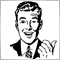8
16 252

### Introduction

Forex trading begins with the study of theoretical basics: earnings strategy, data analysis methods, successful trading models. All novice traders are guided by the same idea — everyone wants to make money. But everyone defines his own priorities, terms, opportunities, goals, etc.

There are several scenarios for the behavior of a novice trader.

• The "All at once" option: most beginners want to earn a lot and quickly. They succumb to the tempting advertising of a magical and flawless strategy, which can be used for very little money or even for free. All that looks fast and easy, although, losing the deposit is also fast and easy.
• The "Education, education and education" option: there are novices who take training responsibly, with no faith in fairy tales. They thoroughly study the laws of the market and trading systems. And that is when trading on a real account starts — but the profit still turns out to be less than expected by the textbooks. How does this happen and what to do next?

Once in the first situation, most neophytes are forever disappointed in working in financial markets. Novices from the second scenario continue studying the theory and their practical strategies.

• One group wants to try out a studied short-term strategy. But if its members work full time, they are left only with the night hours, because the market is closed on the weekends.
• The second category of traders works using medium-term or long-term strategies. They definitely do not want to spend a whole year refining their strategy on a demo account.

Naturally, you might wonder: if there is a history chart where any strategy can be tested quickly and effectively, why are such difficulties needed? In practice, however, this does not always work: it often happens that a strategy with splendid backtest results works very poorly in the "live" market for some reason. At any rate, it is better to learn trading in systems more or less close to the reality. For instance, market emulators are quite sufficient (such programs can be bought on the Internet).

In this article, I want to discuss my own implementation of such a system in MetaTrader 5. I have written the "Mini Market Emulator" indicator with a limited functionality compared to the full version of the terminal. It is designed for theoretical verification of strategies.

### Application features

The application has its own control panel, as well as certain buttons of the "parent system" that is, the MetaTrader 5 terminal itself.

Here are the main actions that can be performed by the emulator.

1. Only two orders in different directions can be placed: buy and sell. It also supports setting of stop loss and take profit before setting the order and its volume. Once the order is placed, it can be modified, and its stop levels can be dragged.
2. There are only seven modeling speeds, they can be divided into three groups. The first is "jewelry", it involves modeling based on generation of ticks from the data of minute timeframe, almost like in the Strategy Tester. The second one considers the minute data, builds without generation (this mode is faster but less accurate). The third mode is the fastest: one candle per second is built, regardless of the timeframe.
3. The current trading information is provided: profit, number of points and volume. The data are given for the current and past orders, as well as the general trade from the beginning of emulation.
4. All standard graphical objects present in the terminal are available.
5. All standard timeframes are supported (switched by the buttons of the terminal panel).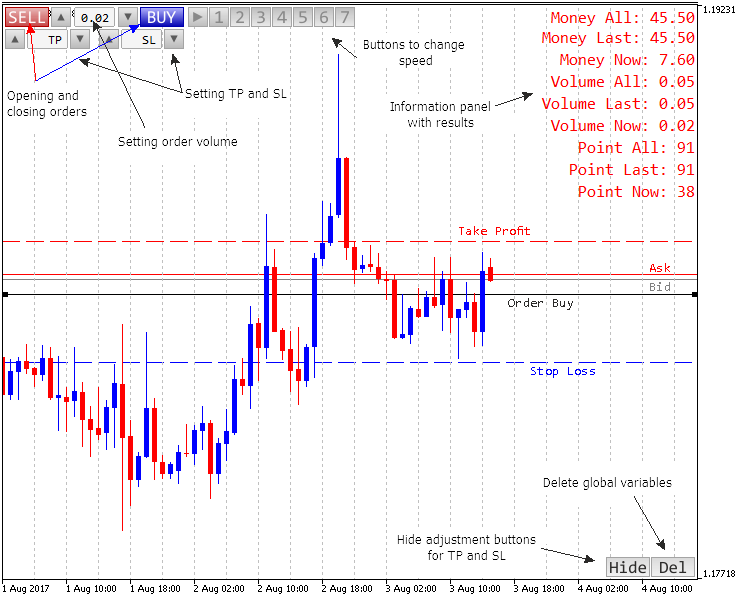Fig. 1. Controls and appearance of the application

### Tick generation system

The principle of the tick generation was taken from the article "The Algorithm of Ticks' Generation within the Strategy Tester of the MetaTrader 5 Terminal". It has been creatively revised and presented as an alternative version.

Two functions are responsible for tick generation — main and auxiliary.

The main function is Tick Generation. It is passed two parameters: the candle itself and an array for the response data (ticks). Then, if all four price levels of the input candle are equal to each other, the volume of ticks is set equal to one tick. This was done to eliminate the possibility of zero division error in case incorrect data are passed.

This is followed by formation of a new candle. If there are 1-3 ticks within the candle, the process of tick generation continues as described in the aforementioned article.

If there are more than 3 ticks, the work becomes more complicated. The passed candle is divided into three unequal parts (the principle of division is provided in the code below, separately for bearish and bullish candles). Then, in case there are no more ticks at the top and bottom, adjustment is made. Next, the control is transferred to the auxiliary function depending on the nature of the candle.

```//+------------------------------------------------------------------+
//| Func Tick Generation                                             |
//+------------------------------------------------------------------+
void func_tick_generation(
MqlRates &rates,      // data on candle
double &tick[]        // dynamic array of ticks
)
{
if(rates.open==rates.close && rates.high==rates.low && rates.open==rates.high){rates.tick_volume=1;}
if(rates.tick_volume<4)// less than four ticks
{
ArrayResize(tick,int(rates.tick_volume));         // resize the array to the number of ticks
if(rates.tick_volume==1)tick=rates.close;      // one tick
if(rates.tick_volume==2)                          // two ticks
{
tick=rates.open;
tick=rates.close;
}
if(rates.tick_volume==3)                          // three ticks
{
tick=rates.open;
tick=rates.close;
if(rates.open==rates.close)                      // went in one direction and returned to the level of Open
{
if(rates.high==rates.open)tick=rates.low;
if(rates.low==rates.open)tick=rates.high;
}
if(rates.close==rates.low && rates.open!=rates.high)tick=rates.high;           // went in one direction, rolled back and broke the level of Open
if(rates.close==rates.high && rates.open!=rates.low)tick=rates.low;
if(rates.open==rates.high && rates.close!=rates.low)tick=rates.low;            // went in one direction, rolled back, but did not break the level of Open
if(rates.open==rates.low && rates.close!=rates.high)tick=rates.high;
if((rates.open==rates.low && rates.close==rates.high) || (rates.open==rates.high && rates.close==rates.low))
{
tick=NormalizeDouble((((rates.high-rates.low)/2)+rates.low),_Digits);       // several points in one direction
}
}
}
if(rates.tick_volume>3)      // more than three ticks
{

// calculate the candle size by points
int candle_up=0;
int candle_down=0;
int candle_centre=0;
if(rates.open>rates.close)
{
candle_up=int(MathRound((rates.high-rates.open)/_Point));
candle_down=int(MathRound((rates.close-rates.low)/_Point));
}
if(rates.open<=rates.close)
{
candle_up=int(MathRound((rates.high-rates.close)/_Point));
candle_down=int(MathRound((rates.open-rates.low)/_Point));
}
candle_centre=int(MathRound((rates.high-rates.low)/_Point));
int candle_all=candle_up+candle_down+candle_centre;      // total length of movement
int point_max=int(MathRound(double(candle_all)/2));      // the maximum possible number of ticks
double share_up=double(candle_up)/double(candle_all);
double share_down=double(candle_down)/double(candle_all);
double share_centre=double(candle_centre)/double(candle_all);

// calculate the number of reference points on each section
char point=0;
if(rates.tick_volume<10)point=char(rates.tick_volume);
else point=10;
if(point>point_max)point=char(point_max);
char point_up=char(MathRound(point*share_up));
char point_down=char(MathRound(point*share_down));
char point_centre=char(MathRound(point*share_centre));

// check for reference points on the selected ranges
if(candle_up>0 && point_up==0)
{point_up=1;point_centre=point_centre-1;}
if(candle_down>0 && point_down==0)
{point_down=1;point_centre=point_centre-1;}

// resize the output array
ArrayResize(tick,11);
char p=0;                     // index of the ticks array (tick[])
tick[p]=rates.open;           // the first tick is equal to the Open price
if(rates.open>rates.close)    // downward
{
func_tick_small(rates.high,1,candle_up,point_up,tick,p);
func_tick_small(rates.low,-1,candle_centre,point_centre,tick,p);
func_tick_small(rates.close,1,candle_down,point_down,tick,p);
ArrayResize(tick,p+1);
}
if(rates.open<=rates.close)   // upward or Doji
{
func_tick_small(rates.low,-1,candle_down,point_down,tick,p);
func_tick_small(rates.high,1,candle_centre,point_centre,tick,p);
func_tick_small(rates.close,-1,candle_up,point_up,tick,p);
ArrayResize(tick,p+1);
}
}
}

```

As the name suggests, the Tick Small function performs a minor generation of ticks. It receives information about the last processed tick, the direction to go (up or down), the required number of steps, the last price, and passes the calculated steps to the above array of ticks. The resulting array contains no more than 11 ticks.

```//+------------------------------------------------------------------+
//| Func Tick Small                                                  |
//+------------------------------------------------------------------+
void func_tick_small(
double end,        // end of movement
char route,        // direction of movement
int candle,        // distance of movement
char point,        // the number of points
double &tick[],    // array of ticks
char&i           // the current index of the array
)
{
if(point==1)
{
i++;
tick[i]=end;
}
if(point>1)
{
double wave_v=(point+1)/2;
double step_v=(candle-1)/MathFloor(wave_v)+1;
step_v=MathFloor(step_v);
for(char p_v=i+1,i_v=i; p_v<i_v+point;)
{
i++;
if(route==1)tick[i]=tick[i-1]+(step_v*_Point);
if(route==-1)tick[i]=tick[i-1]-(step_v*_Point);
p_v++;
if(p_v<i_v+point)
{
i++;
if(route==1)tick[i]=tick[i-1]-_Point;
if(route==-1) tick[i]=tick[i-1]+_Point;
}
p_v++;
}
if(NormalizeDouble(tick[i],_Digits)!=NormalizeDouble(end,_Digits))
{
i++;
tick[i]=end;
}
}
}

```

This is, so to say, the heart of the entire "jewelry" modeling (the conclusion explains why it is called "jewelry"). Now let us move on to the essence of system interaction.

### Interaction and data exchange

The code of the system seems confusing at first glance. Functions are not entirely consistent, their calls from different parts of the program are possible. It turned out like this, because the system has to interact not only with the user, but also with the terminal. Here is an approximate scheme of those interactions (Fig. 2):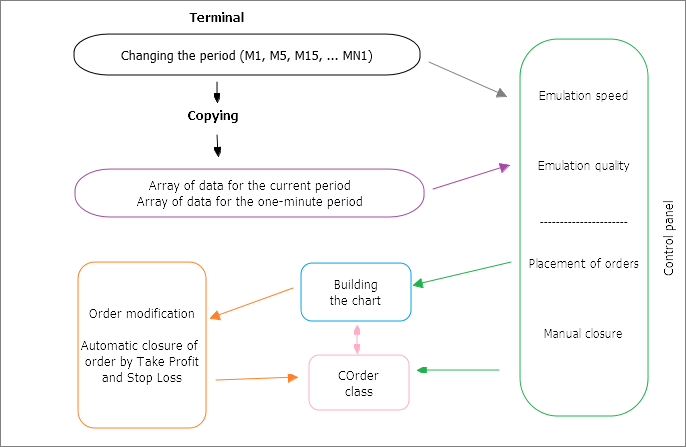Fig. 2. Schema of interactions in the application

To reduce the number of control objects in the indicator window, the mechanism for switching periods was borrowed from the terminal shell. But since the application is reinitialized when switching the period and all variables on the local and global scopes are overwritten, the array of data is copied every time a switching occurs. In particular, the data of two periods are copied — M1 and the selected one. Parameters for subsequent processing of these data are selected on the panel: the speed and quality of simulation ("jewelry" or simple fast method). Once everything is ready, modeling of the chart begins.

The control panel is convenient for placing orders and deleting them. To do this, the program refers to the "COrder" class. This class is also used for managing orders as the chart is built.

As mentioned above, if the chart period changes, the indicator restarts. Accordingly, global variables of the client terminal are used to provide communication throughout the entire structure of the application. Unlike the conventional global variables, they are stored for a longer time (4 weeks) and are tolerant to restarts. The only drawback is their data type, which is limited to double. But in general, this is much more convenient than creating a new file, writing and reading it every time.

Let us move on to the code of the interaction elements directly.

### Implementation in code

Beginning of the code

First come the standard procedures for declaring variables. Then the OnInit() function initializes the buffers, draws the interface of the control panel, calculates the offset from the beginning of the emulation. The offset is required to make sure the simulation does not start with an empty chart, but with a certain history to begin checking the strategy right away.

The data arrays are copied and the main connective variable is read (named time_end) here as well. It indicates the time at which the simulation stopped:

```//--- set the time up to which the indicator was drawn
if(GlobalVariableCheck(time_end))end_time_indicator=datetime(GlobalVariableGet(time_end));

```

This way the indicator always "knows" where it stopped. The OnInit() function ends with a timer call, which, in fact, gives the command to output a new tick or to form an entire candle (depending on the speed).

Timer function

The state of the "play" button on the control panel is checked at the beginning of the function. If it is pressed, the further code is executed.

First, it determines the indicator bar where the simulation stopped (relative to the current time). The last simulation time 'end_time_indicator' and the current time are taken as endpoints. The data are recalculated every second, as the chart is moving constantly (except for Saturday and Sunday) and it is not synchronized in time. Thus, the chart is dynamically tracked and moved by the ChartNavigate() function.

After that, the variables 'number_now_rates', 'bars_now_rates', 'all_bars_indicator' are calculated. The time is checked afterwards. If it has not run out according to the input parameters, modeling is performed using the func_merger() function. Next, the current positions and their profitability are checked, with the values recorded in the global variables and output in the information block of the indicator.

The "COrder" class is also called here, namely, its parts responsible for automatic deletion of orders as a result of user actions (position.Delete) or the activation of stop levels (position.Check).

```//+------------------------------------------------------------------+
//| Timer function                                                   |
//+------------------------------------------------------------------+
void OnTimer()
{
//---
if(button_play)
{
end_bar_indicator=Bars(_Symbol,_Period,end_time_indicator,TimeCurrent());      // the number of bars from the earliest to the current
ChartNavigate(0,CHART_END,-end_bar_indicator);                                 // move the chart (indicator) to the currently modeled bar
number_now_rates=(Bars(_Symbol,_Period,real_start,end_time_indicator)-1);      // the bar currently used for modeling
bars_now_rates=(Bars(_Symbol,_Period,real_start,stop)-1);                      // the number of bars used from history for the current period
all_bars_indicator=(Bars(_Symbol,_Period,real_start,TimeCurrent()))-1;         // the number of bars from the beginning of the simulation to the current time

if(end_time_indicator<stop)                                                    // checking the simulation time
{
func_merger();
ObjectSetDouble(0,line_bid,OBJPROP_PRICE,price_bid_now);

//--- the current values for orders
int point_now=0;
double vol_now=0;
double money_now=0;
{
double m_now=p_now*v_now*10;
point_now+=p_now;
vol_now+=v_now;
money_now+=m_now;
}
if(ObjectFind(0,order_sell)>=0 && GlobalVariableGet(order_sell)>0)           // a sell order is present
{
double v_now=GlobalVariableGet(vol_sell);
double m_now=p_now*v_now*10;
point_now+=p_now;
vol_now+=v_now;
money_now+=m_now;
}
GlobalVariableSet(info_point_now,point_now);
GlobalVariableSet(info_vol_now,vol_now);
GlobalVariableSet(info_money_now,money_now);
}

COrder position;    //object of the "COrder" class
GlobalVariableGet(order_sell),GlobalVariableGet(tp_sell),GlobalVariableGet(sl_sell));

func_info_print("Money All: ",info_money_all,2);
func_info_print("Money Last: ",info_money_last,2);
func_info_print("Money Now: ",info_money_now,2);
func_info_print("Volume All: ",info_vol_all,2);
func_info_print("Volume Last: ",info_vol_last,2);
func_info_print("Volume Now: ",info_vol_now,2);
func_info_print("Point All: ",info_point_all,0);
func_info_print("Point Last: ",info_point_last,0);
func_info_print("Point Now: ",info_point_now,0);

position.Modify();
}
//--- managing the Hide button
char x=char(GlobalVariableGet("hide"));
if(x==1)
{
ObjectSetInteger(0,"20",OBJPROP_STATE,false);
ObjectSetInteger(0,"14",OBJPROP_YDISTANCE,24);
ObjectSetInteger(0,"15",OBJPROP_YDISTANCE,24);
ObjectSetInteger(0,"16",OBJPROP_YDISTANCE,24);
ObjectSetInteger(0,"17",OBJPROP_YDISTANCE,24);
ObjectSetInteger(0,"18",OBJPROP_YDISTANCE,24);
ObjectSetInteger(0,"19",OBJPROP_YDISTANCE,24);
}
if(x==2)
{
ObjectSetInteger(0,"20",OBJPROP_STATE,true);
ObjectSetInteger(0,"14",OBJPROP_YDISTANCE,-24);
ObjectSetInteger(0,"15",OBJPROP_YDISTANCE,-24);
ObjectSetInteger(0,"16",OBJPROP_YDISTANCE,-24);
ObjectSetInteger(0,"17",OBJPROP_YDISTANCE,-24);
ObjectSetInteger(0,"18",OBJPROP_YDISTANCE,-24);
ObjectSetInteger(0,"19",OBJPROP_YDISTANCE,-24);
}
}
```

The COrder class

This class contains the functions for opening and closing positions, modifying and checking the current state of orders (management of their take profit and stop loss levels).

Let us start with placement of orders using Placed. The order type (buy or sell) is selected by means of the switch operator, the data are stored in a global variable (order_buy or order_sell). If m_take_profit and m_stop_loss have been previously defined, store them in the corresponding global variables and draw their lines on the chart. The lines are set by the Line function of this class.

```//+------------------------------------------------------------------+
//| Class COrder                                                     |
//+------------------------------------------------------------------+
class COrder
{
public:
void Placed(
char m_type,// order type (1-buy, 2-sell)
double m_price_bid, // Bid price
int m_take_profit,// points to take profit
int m_stop_loss // points to stop loss
)
{
switch(m_type)
{
case 1:
{
if(m_take_profit>0)
{
}
if(m_stop_loss>0)
{
}
}
break;
case 2:
{
GlobalVariableSet(order_sell,m_price_bid);
Line(GlobalVariableGet(order_sell),order_sell,col_sell,STYLE_SOLID,1,true);
if(m_take_profit>0)
{
GlobalVariableSet(tp_sell,(m_price_bid-(_Point*m_take_profit)));
Line(GlobalVariableGet(tp_sell),tp_sell,col_tp,STYLE_DASH,1,true);
}
if(m_stop_loss>0)
{
GlobalVariableSet(sl_sell,(m_price_bid+(_Point*m_stop_loss)));
Line(GlobalVariableGet(sl_sell),sl_sell,col_sl,STYLE_DASH,1,true);
}
}
break;
}
}
```

Next comes the Delete function for deleting orders. Again, the switch operator selects one of the three options — automatic deletion, buy or sell. In this case, automatic deletion is a situation, where an order is deleted by deleting its line from the chart.

This is done by the Small_del_buy and Small_del_sell auxiliary functions of the class.

``` void Delete(
double m_price_bid,      // Bid price
char m_del_manual        // deletion type (-1 - auto, 1 - buy, 2 - sell)
)
{
switch(m_del_manual)
{
case(-1):
if(ObjectFind(0,order_sell)<0 && GlobalVariableGet(order_sell)>0)
break;
case 1:
{
}
break;
case 2:
if(ObjectFind(0,order_sell)>=0)
{
ObjectDelete(0,order_sell);
}
break;
}
}

```

Let us consider one of them — Small_del_sell.

Check for the take profit and stop loss lines. If they are present, delete them. Then the order_sell global variable is zeroed. This will be needed later, in case the global variables are used to check for presence of orders.

The information on the profit of orders is also stored in the global variables (info_point_last, info_vol_last, info_money_last). This is done by small_concatenation (similar to the += operator, but with global variables). Summarize the profit (volume) and also store it in global variables (info_point_all, info_vol_all, info_money_all).

```void Small_del_sell(double m_price_ask)
{
if(ObjectFind(0,tp_sell)>=0)ObjectDelete(0,tp_sell);       // delete the take profit line
if(ObjectFind(0,sl_sell)>=0)ObjectDelete(0,sl_sell);      // delete the stop loss line
GlobalVariableSet(order_sell,0);                           // zero the variable for the price of the placed order
GlobalVariableSet(info_vol_last,GlobalVariableGet(vol_sell));
GlobalVariableSet(vol_sell,0);
GlobalVariableSet(info_point_last,point_plus);
GlobalVariableSet(info_money_last,(GlobalVariableGet(info_point_last)*GlobalVariableGet(info_vol_last)*10));
Small_concatenation(info_point_all,info_point_last);
Small_concatenation(info_vol_all,info_vol_last);
Small_concatenation(info_money_all,info_money_last);
}
```

Modification of an order is done by changing its location with the mouse. There are two ways to do this. The first is attempting to drag the order opening line. In this case, new take profit and stop loss lines are plotted, depending on the movement direction and order type. The Small_mod function is also implemented in the COrder class. Its input parameters are the object name, permission to move the object and order type.

At the beginning of the Small_mod function, the presence of object is checked. Then, if moving the take profit/stop loss lines is allowed, the change in the price is stored in a global variable. If moving (buy and sell lines) is prohibited, then, depending on the order type, a new take profit or stop loss line appears on the new location of the line, and the order line returns to its place.

``` void Small_mod(string m_name,      // name of the object and global variable
bool m_mode,                        // permission to change position
char m_type                         // 1 — buy, 2 — sell
)
{
if(ObjectFind(0,m_name)>=0)
{
double price_obj_double=ObjectGetDouble(0,m_name,OBJPROP_PRICE);
int price_obj=int(price_obj_double*dig_pow);
double price_glo_double=GlobalVariableGet(m_name);
int price_glo=int(price_glo_double*dig_pow);
if(price_obj!=price_glo && m_mode==true)
{
GlobalVariableSet(m_name,(double(price_obj)/double(dig_pow)));
}
if(price_obj!=price_glo && m_mode==false)
{
switch(m_type)
{
if(price_obj>price_glo)          // TP
{
}
if(price_obj<price_glo)          // SL
{
}
break;
case 2:                        // order sell
if(price_obj>price_glo)         // SL
{
GlobalVariableSet(sl_sell,(double(price_obj)/double(dig_pow)));
Line(GlobalVariableGet(sl_sell),sl_sell,col_sl,STYLE_DASH,1,true);
}
if(price_obj<price_glo)         // TP
{
GlobalVariableSet(tp_sell,(double(price_obj)/double(dig_pow)));
Line(GlobalVariableGet(tp_sell),tp_sell,col_tp,STYLE_DASH,1,true);
}
break;
}
ObjectSetDouble(0,m_name,OBJPROP_PRICE,(double(price_glo)/double(dig_pow)));
}
}
}

```

During the modeling of the chart, orders are constantly checked by the Check function of the COrder class. The function is passed all global variables that store information on orders. There is also a separate global variable that contains the information about the time of the last call. This allows each call to check the entire price range (one-minute timeframe) in the interval between the last call to the function and the current chart drawing time.

In case the price reaches one of the stop lines or breaks it during this time, the control is passed to the function for deleting orders (the Delete function in the COrder class).

``` void Check(
datetime m_time,
double m_price_sell,
double m_price_tp_sell,
double m_price_sl_sell
)
{
int start_of_z=0;
int end_of_z=0;
datetime time_end_check=datetime(GlobalVariableGet(time_end_order_check));
if(time_end_check<=0){time_end_check=m_time;}
GlobalVariableSet(time_end_order_check,m_time);
start_of_z=Bars(_Symbol,PERIOD_M1,real_start,time_end_check);
end_of_z=Bars(_Symbol,PERIOD_M1,real_start,m_time);
for(int z=start_of_z; z<end_of_z; z++)
{
COrder del;
double p_bid_high=period_m1[z].high;
double p_bid_low=period_m1[z].low;
{
{
{del.Delete(m_price_tp_buy,0,1);}                              // close at the TP price
}
{
{del.Delete(m_price_sl_buy,0,1);}                              // close at the SL price
}
}
if(m_price_sell>0)                                                   // there is a SELL order
{
if(ObjectFind(0,tp_sell)>=0)
{
{del.Delete(0,m_price_sl_sell,2);}                             // close at the SL price
}
if(ObjectFind(0,sl_sell)>=0)
{
{del.Delete(0,m_price_tp_sell,2);}                             // close at the TP price
}
}
}
}

```

This concludes the main functions of the class. Let us examine the functions directly responsible for drawing the candles on the chart.

The func_filling() function

Since switching the period reinitializes the indicator, it is necessary to refill the chart and place the past candles up to the current candle time (so-called "tail"). This function is used before a new candle is generated as well, which allows normalizing the "tail" of the chart and increasing the display accuracy.

The function is passed an array of data of the current period, the current display time, the number of all candles and the currently drawn candle. Once executed, the function returns the opening time of the last displayed candle and the opening time of the candle that follows it. The indicator array is also filled, and the function completion flag 'work_status' is returned.

The function uses a 'for' loop to fill the entire indicator buffer previously displayed up to the drawn candle, and also the price values of the currently drawn candle (usually equal to the Open prices).

```//+------------------------------------------------------------------+
//| Func Filling |
//+------------------------------------------------------------------+
void func_filling(MqlRates &input_rates[],                // input data (of the current period) to fill
datetime input_end_time_indicator,      // the current time of the indicator
int input_all_bars_indicator,           // the number of all bars of the indicator
datetime &output_time_end_filling,      // the opening time of the last bar
datetime &output_time_next_filling,     // the opening time of the next bar
int input_end_bar_indicator,            // the current (drawn) bar of the indicator
double &output_o[],
double &output_h[],
double &output_l[],
double &output_c[],
double &output_col[],
char &work_status)                      // operation status
{
if(work_status==1)
{
int stopped_rates_bar;
for(int x=input_all_bars_indicator,y=0;x>0;x--,y++)
{
if(input_rates[y].time<input_end_time_indicator)
{
output_o[x]=input_rates[y].open;
output_h[x]=input_rates[y].high;
output_l[x]=input_rates[y].low;
output_c[x]=input_rates[y].close;
if(output_o[x]>output_c[x])output_col[x]=0;
else output_col[x]=1;
output_time_end_filling=input_rates[y].time;
output_time_next_filling=input_rates[y+1].time;
input_end_bar_indicator=x;
stopped_rates_bar=y;
}
else break;
}
output_o[input_end_bar_indicator]=input_rates[stopped_rates_bar].open;
output_h[input_end_bar_indicator]=output_o[input_end_bar_indicator];
output_l[input_end_bar_indicator]=output_o[input_end_bar_indicator];
output_c[input_end_bar_indicator]=output_o[input_end_bar_indicator];
work_status=-1;
}
}
```

Once executed, the control is transferred to one of the three functions for drawing the current candle. Let us consider them in order starting from the fastest one.

The func_candle_per_seconds() function for drawing the candle every second

Unlike the other two functions, here the control is not transferred to other functions before the indicator is reloaded or the chart drawing speed is changed. Every new call occurs every second by timer, and during this time, the current candle is drawn (filled with data). First, the data are copied from the passed array to the current candle, then the initial data are passed to the next candle. At the very end, the function passes the time the last candle was formed.

The function described above is responsible for the "seventh speed" of candle generation (see the control panel).

```//+------------------------------------------------------------------+
//| Func Candle Per Seconds                                          |
//+------------------------------------------------------------------+
void func_candle_per_seconds(MqlRates &input_rates[],
datetime &input_end_time_indicator,
int input_bars_now_rates,
int input_number_now_rates,
int &input_end_bar_indicator,
double &output_o[],
double &output_h[],
double &output_l[],
double &output_c[],
double &output_col[],
char &work_status)
{
if(work_status==-1)
{
if(input_number_now_rates<input_bars_now_rates)
{
if(input_number_now_rates!=0)
{
output_o[input_end_bar_indicator]=input_rates[input_number_now_rates-1].open;
output_h[input_end_bar_indicator]=input_rates[input_number_now_rates-1].high;
output_l[input_end_bar_indicator]=input_rates[input_number_now_rates-1].low;
output_c[input_end_bar_indicator]=input_rates[input_number_now_rates-1].close;
if(output_o[input_end_bar_indicator]>output_c[input_end_bar_indicator])output_col[input_end_bar_indicator]=0;
else output_col[input_end_bar_indicator]=1;
}
input_end_bar_indicator--;
output_o[input_end_bar_indicator]=input_rates[input_number_now_rates].open;
output_h[input_end_bar_indicator]=input_rates[input_number_now_rates].high;
output_l[input_end_bar_indicator]=input_rates[input_number_now_rates].low;
output_c[input_end_bar_indicator]=input_rates[input_number_now_rates].close;
if(output_o[input_end_bar_indicator]>output_c[input_end_bar_indicator])output_col[input_end_bar_indicator]=0;
else output_col[input_end_bar_indicator]=1;
input_end_time_indicator=input_rates[input_number_now_rates+1].time;
}
}
}
```

The following two functions are very similar to each other. One of them build candles by time, despite the ticks. The second one ("jewelry") uses the tick generator described at the beginning of the article for a more complete emulation of the market.

The func_of_form_candle() for building candles

The input parameters are the same as before (OHLC). As for functionality, everything is quite simple. The prices are copied from the M1 timeframe data to the current candle in a cycle, starting from the time received from the func_filling() function. It turns out that by changing the time, a candle is gradually formed. Speeds from the second to sixth are constructed this way (see the control panel). After the time reaches the moment of the candle completion on the current timeframe, the 'work_status' flag is changed, so that the next execution of the timer invokes the func_filling() function again.

```//+------------------------------------------------------------------+
//| Func Of Form Candle                                              |
//+------------------------------------------------------------------+
void func_of_form_candle(MqlRates &input_rates[],
int input_bars,
datetime &input_time_end_filling,
datetime &input_end_time_indicator,
datetime &input_time_next_filling,
int input_end_bar_indicator,
double &output_o[],
double &output_h[],
double &output_l[],
double &output_c[],
double &output_col[],
char &work_status)
{
if(work_status==-1)
{
int start_of_z=0;
int end_of_z=0;
start_of_z=Bars(_Symbol,PERIOD_M1,real_start,input_time_end_filling);
end_of_z=Bars(_Symbol,PERIOD_M1,real_start,input_end_time_indicator);
for(int z=start_of_z; z<end_of_z; z++)
{
output_c[input_end_bar_indicator]=input_rates[z].close;
if(output_h[input_end_bar_indicator]<input_rates[z].high)output_h[input_end_bar_indicator]=input_rates[z].high;
if(output_l[input_end_bar_indicator]>input_rates[z].low)output_l[input_end_bar_indicator]=input_rates[z].low;
if(output_o[input_end_bar_indicator]>output_c[input_end_bar_indicator])output_col[input_end_bar_indicator]=0;
else output_col[input_end_bar_indicator]=1;
}
if(input_end_time_indicator>=input_time_next_filling)work_status=1;
}
}
```

Let us now move on to the function, which is able to form a candle that is as close to the market as possible.

The func_of_form_jeweler_candle() function for "jewelry" simulation of candles

At the beginning of the function, everything happens as in the previous version. The data of the minute timeframe completely fill the current candle, except for the last minute. Its data are passed to the func_tick_generation() function for generating ticks, which is described at the beginning of the article. With each call to the function, the received array of ticks is gradually passed as the current candle Close price, taking into account the adjustment for "shadows". When the "ticks" of the array are over, the process is repeated.

```//+------------------------------------------------------------------+
//| Func Of Form Jeweler Candle                                      |
//+------------------------------------------------------------------+
void func_of_form_jeweler_candle(MqlRates &input_rates[],                    // information for generating ticks
int input_bars,                             // size of the information array
datetime &input_time_end_filling,           // end time of quick fill
datetime &input_end_time_indicator,         // the last simulation time of the indicator
datetime &input_time_next_filling,          // time remaining until a full bar of the current timeframe is completely formed
int input_end_bar_indicator,                // the currently drawn bar of the indicator
double &output_o[],
double &output_h[],
double &output_l[],
double &output_c[],
double &output_col[],
char &work_status                           // operation end type (command for the quick fill function)
)
{
if(work_status==-1)
{
int start_of_z=0;
int current_of_z=0;
start_of_z=Bars(_Symbol,PERIOD_M1,real_start,input_time_end_filling)-1;
current_of_z=Bars(_Symbol,PERIOD_M1,real_start,input_end_time_indicator)-1;
if(start_of_z<current_of_z-1)
{
for(int z=start_of_z; z<current_of_z-1; z++)
{
output_c[input_end_bar_indicator]=input_rates[z].close;
if(output_h[input_end_bar_indicator]<input_rates[z].high)output_h[input_end_bar_indicator]=input_rates[z].high;
if(output_l[input_end_bar_indicator]>input_rates[z].low)output_l[input_end_bar_indicator]=input_rates[z].low;
if(output_o[input_end_bar_indicator]>output_c[input_end_bar_indicator])output_col[input_end_bar_indicator]=0;
else output_col[input_end_bar_indicator]=1;
}
input_end_time_indicator=input_rates[current_of_z].time;
}
// get the ticks in the array
static int x=0;                   // array counter and start flag
static double tick_current[];
static int tick_current_size=0;
if(x==0)
{
func_tick_generation(input_rates[current_of_z-1],tick_current);
tick_current_size=ArraySize(tick_current);
if(output_h[input_end_bar_indicator]==0)
{output_h[input_end_bar_indicator]=tick_current[x];}
if(output_l[input_end_bar_indicator]==0)
{output_l[input_end_bar_indicator]=tick_current[x];}
output_c[input_end_bar_indicator]=tick_current[x];
}
if(x<tick_current_size)
{
output_c[input_end_bar_indicator]=tick_current[x];
if(tick_current[x]>output_h[input_end_bar_indicator])
{output_h[input_end_bar_indicator]=tick_current[x];}
if(tick_current[x]<output_l[input_end_bar_indicator])
{output_l[input_end_bar_indicator]=tick_current[x];}
if(output_o[input_end_bar_indicator]>output_c[input_end_bar_indicator])output_col[input_end_bar_indicator]=0;
else output_col[input_end_bar_indicator]=1;
x++;
}
else
{
input_end_time_indicator=input_rates[current_of_z+1].time;
x=0;
tick_current_size=0;
ArrayFree(tick_current);
}
if(input_end_time_indicator>input_time_next_filling)
{work_status=1;}
}
}
```

All three functions for generating candles are combined in the Merger function.

The func_merger() function for combined simulation

The function used in the work is determined depending on the speed selected by the switch operator. The function has three types. Any case starts with the func_filling() function, then the control is passed to one of the three candle generation functions: func_of_form_jeweler_candle(), func_of_form_candle() or func_candle_per_seconds(). The time is recalculated on each pass on the second to sixth speeds, inclusively. The func_calc_time() function calculates the required part of the current timeframe and adds it to the current time. The Bid price is taken from the Close price of the current candle, and Ask is calculated based on spread data received from the server.

```//+------------------------------------------------------------------+
//| Func Merger                                                      |
//+------------------------------------------------------------------+
void func_merger()
{
switch(button_speed)
{
case 1:
{
func_filling(period_array,end_time_indicator,all_bars_indicator,time_open_end_rates,time_open_next_rates,end_bar_indicator,TST_C_O,TST_C_H,TST_C_L,TST_C_C,TST_C_Col,status);
func_of_form_jeweler_candle(period_m1,bars_m1,time_open_end_rates,end_time_indicator,time_open_next_rates,end_bar_indicator,TST_C_O,TST_C_H,TST_C_L,TST_C_C,TST_C_Col,status);
price_bid_now=TST_C_C[end_bar_indicator];
}
break;
case 2:
{
func_filling(period_array,end_time_indicator,all_bars_indicator,time_open_end_rates,time_open_next_rates,end_bar_indicator,TST_C_O,TST_C_H,TST_C_L,TST_C_C,TST_C_Col,status);
func_of_form_candle(period_m1,bars_m1,time_open_end_rates,end_time_indicator,time_open_next_rates,end_bar_indicator,TST_C_O,TST_C_H,TST_C_L,TST_C_C,TST_C_Col,status);
price_bid_now=TST_C_C[end_bar_indicator];
end_time_indicator+=func_calc_time(time_open_end_rates,time_open_next_rates,13);
}
break;
case 3:
{
...
end_time_indicator+=func_calc_time(time_open_end_rates,time_open_next_rates,11);
}
break;
case 4:
{
...
end_time_indicator+=func_calc_time(time_open_end_rates,time_open_next_rates,9);
}
break;
case 5:
{
...
end_time_indicator+=func_calc_time(time_open_end_rates,time_open_next_rates,7);
}
break;
case 6:
{
...
end_time_indicator+=func_calc_time(time_open_end_rates,time_open_next_rates,5);
}
break;
case 7:
{
func_filling(period_array,end_time_indicator,all_bars_indicator,time_open_end_rates,time_open_next_rates,end_bar_indicator,TST_C_O,TST_C_H,TST_C_L,TST_C_C,TST_C_Col,status);
func_candle_per_seconds(period_array,end_time_indicator,bars_now_rates,number_now_rates,end_bar_indicator,TST_C_O,TST_C_H,TST_C_L,TST_C_C,TST_C_Col,status);
price_bid_now=TST_C_C[end_bar_indicator];
}
break;
}
}
```

### Possible uses

I suggest using this indicator for testing new ideas and trading strategies, modeling the behavior of a novice trader in a particular situation, practicing market entries and exits. This primarily concerns technical tools: for example, this indicator can be used for plotting the Elliott waves, channels or for testing the work of support/resistance lines.

Example of the indicator operation:

### Conclusion

Now I will reveal the secret: why was one of the generation types called "jewelry" after all?

It is simple. While developing this application, I came to the conclusion that such smooth and accurate modeling is not necessary for testing most strategies. Therefore, it is a kind of luxury, a piece of jewelry. These ticks simulate the fluctuations almost comparable to the spread and have little impact on the flow of the strategy, much less considering the test speed. It is unlikely for anyone to waste several days to catch an entry point, when it is possible to rewind to the next convenient point.

As for the code, the possibility of various failures is not excluded. However, this should not affect the analysis of the strategy as a whole. After all, all the basic actions are stored in the global variables, and it is possible to simply reload the timeframe or the terminal (without closing the indicator window), and then continue the further emulation.

Many auxiliary functions have been omitted in the description of the code. They are straightforward or are already explained in the documentation. In any case, feel free to ask questions if there is anything you do not understand. As always, any comments are highly appreciated.

Translated from Russian by MetaQuotes Software Corp.
Original article: https://www.mql5.com/ru/articles/3965

Attached files |
STSv1.1.ex5 (120.96 KB)
STSv1.1.mq5 (127.38 KB)
for_STS.zip (13.39 KB)
Last comments | Go to discussion (8)| 13 Dec 2018 at 17:15
henry2896:
hi, something don't work i can trade here

Hey. I did not check the performance on this dealing center. But it should work. Perhaps indicator are typing errors there. And the screenshot shows that the indicator didn’t turn around as it should ... Try to trim the beginning of the construction, for example, in the region of a couple of months from the current date, to test...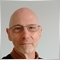| 21 Dec 2018 at 14:38

Hello,

I tested this indicator and I have the following error.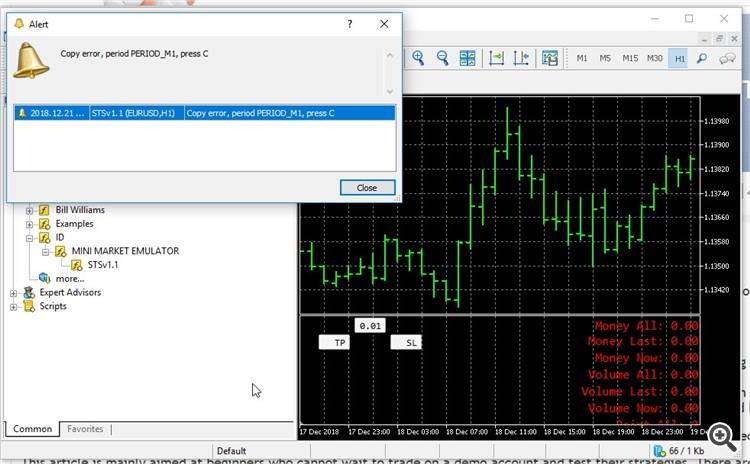| 14 Jan 2019 at 19:11
Pierre Rougier:

Hello,

I tested this indicator and I have the following error.

This question has already been raised (#1), the answer is the following:

Hello. The indicator copies historical data, but they are not always loaded in the MT5. Try using the date closer to the current date, for example 01/08/2018. If the problem goes away, then the point is this.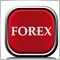| 22 Mar 2019 at 19:54
Is there any way to have the MT4 version?| 1 Apr 2019 at 21:44
Mike Mohebbi:
Is there any way to have the MT4 version?

I did not write on MT4. But maybe someone will write.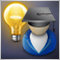Fuzzy Logic in trading strategies

The article considers an example of applying the fuzzy logic to build a simple trading system, using the Fuzzy library. Variants for improving the system by combining fuzzy logic, genetic algorithms and neural networks are proposed.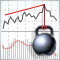A New Approach to Interpreting Classic and Hidden Divergence

The article considers the classic method for divergence construction and provides an additional divergence interpretation method. A trading strategy was developed based on this new interpretation method. This strategy is also described in the article.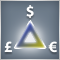Triangular arbitrage

The article deals with the popular trading method - triangular arbitrage. Here we analyze the topic in as much detail as possible, consider the positive and negative aspects of the strategy and develop the ready-made Expert Advisor code.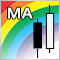Comparing different types of moving averages in trading

This article deals with seven types of moving averages (MA) and a trading strategy to work with them. We also test and compare various MAs at a single trading strategy and evaluate the efficiency of each moving average compared to others.